# Random Variables and Stochastic Processes 0903720 Lecture14 Dr

• Slides: 36
Download presentation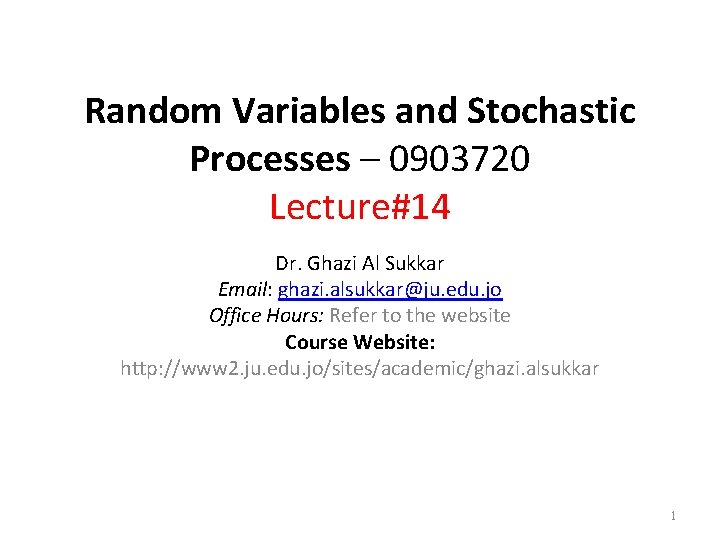Random Variables and Stochastic Processes – 0903720 Lecture#14 Dr. Ghazi Al Sukkar Email: ghazi. [email protected] edu. jo Office Hours: Refer to the website Course Website: http: //www 2. ju. edu. jo/sites/academic/ghazi. alsukkar 1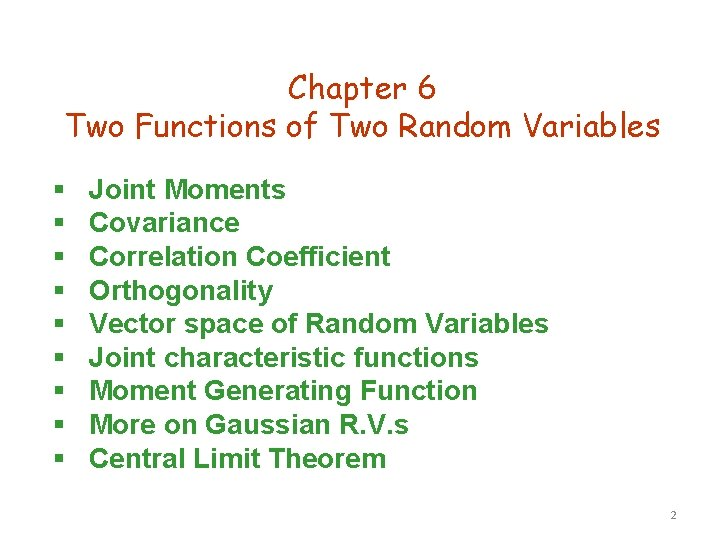Chapter 6 Two Functions of Two Random Variables § § § § § Joint Moments Covariance Correlation Coefficient Orthogonality Vector space of Random Variables Joint characteristic functions Moment Generating Function More on Gaussian R. V. s Central Limit Theorem 2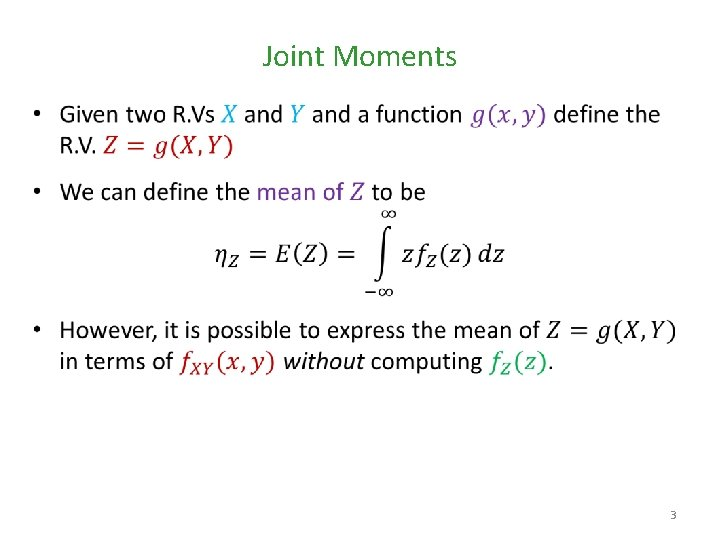Joint Moments • 3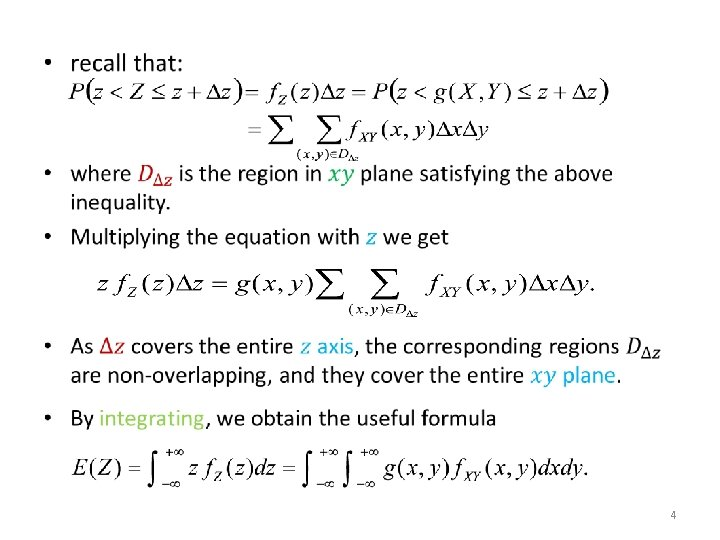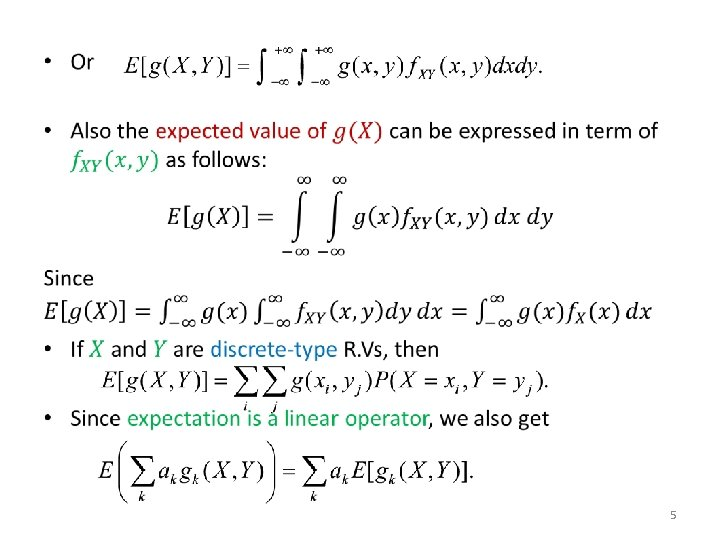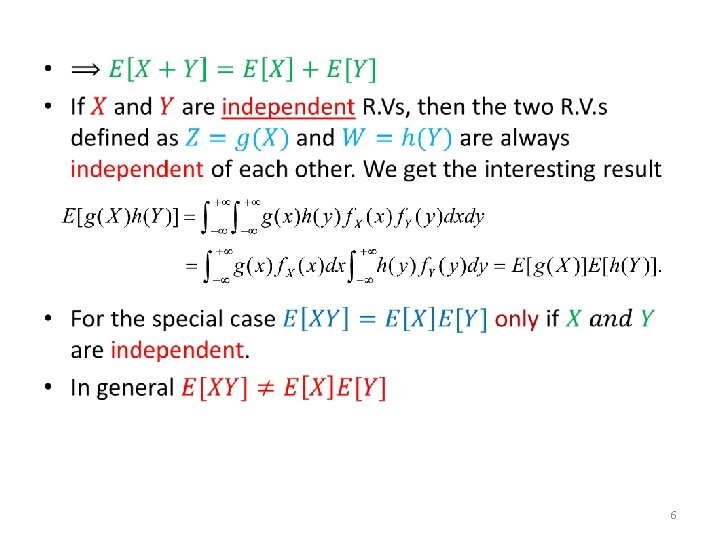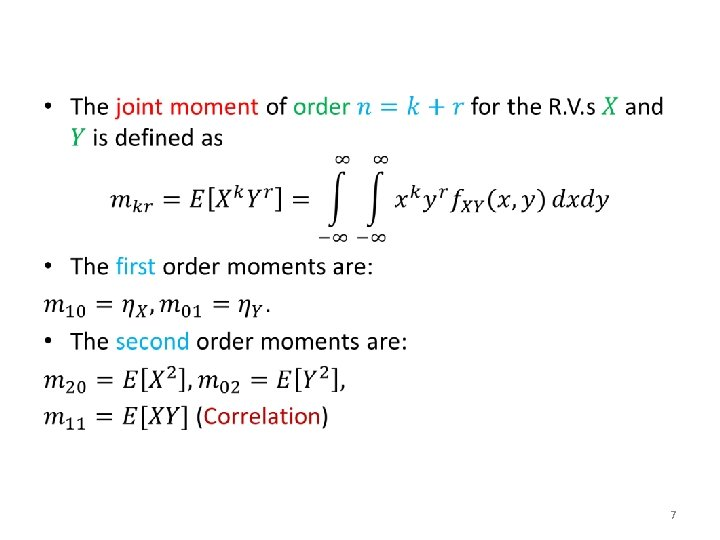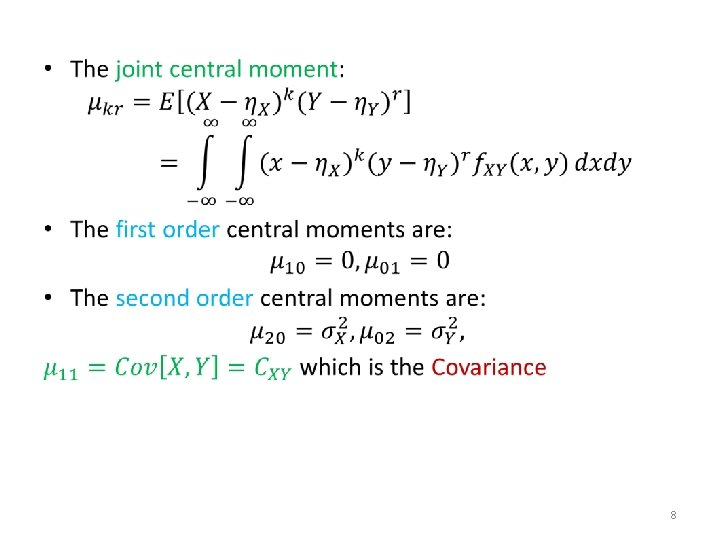Covariance • 9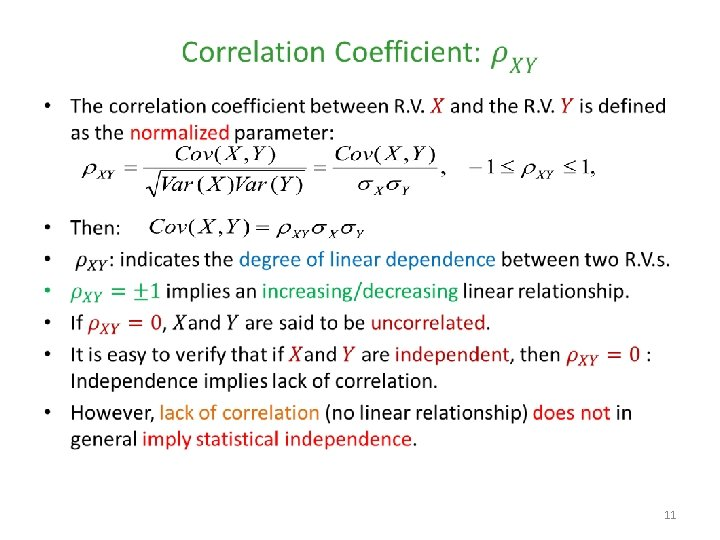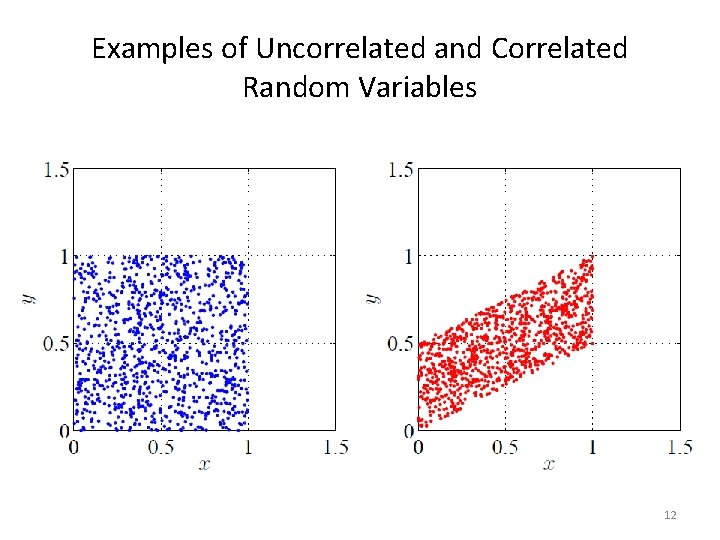Examples of Uncorrelated and Correlated Random Variables 12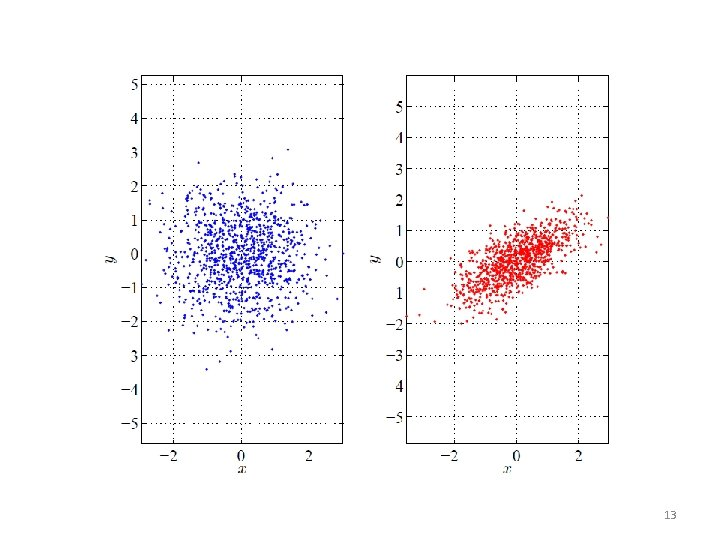13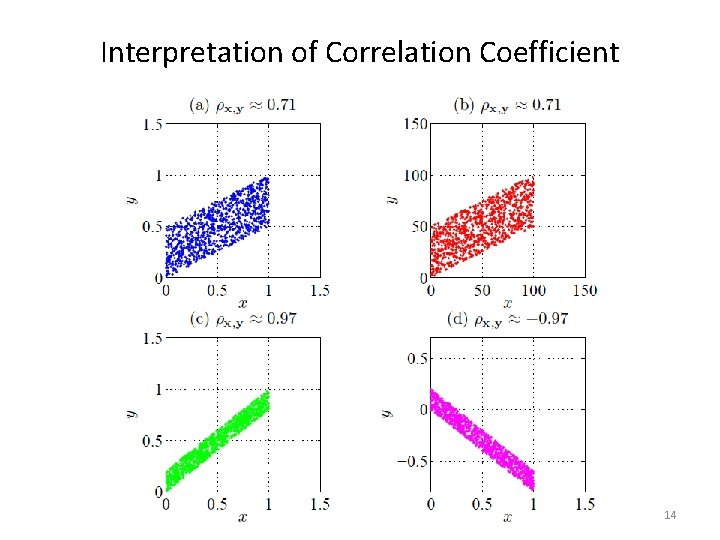Interpretation of Correlation Coefficient 14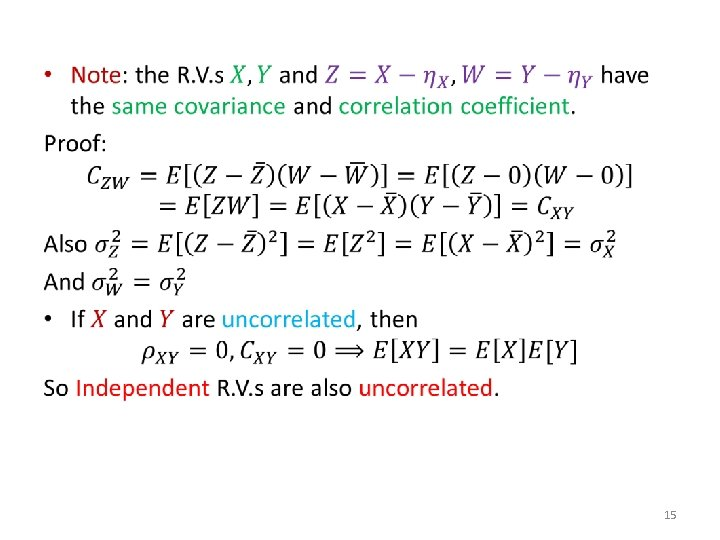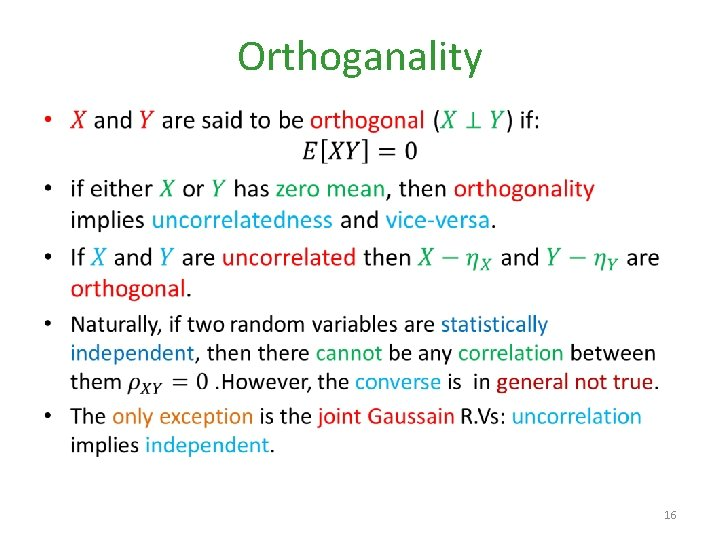Orthoganality • 16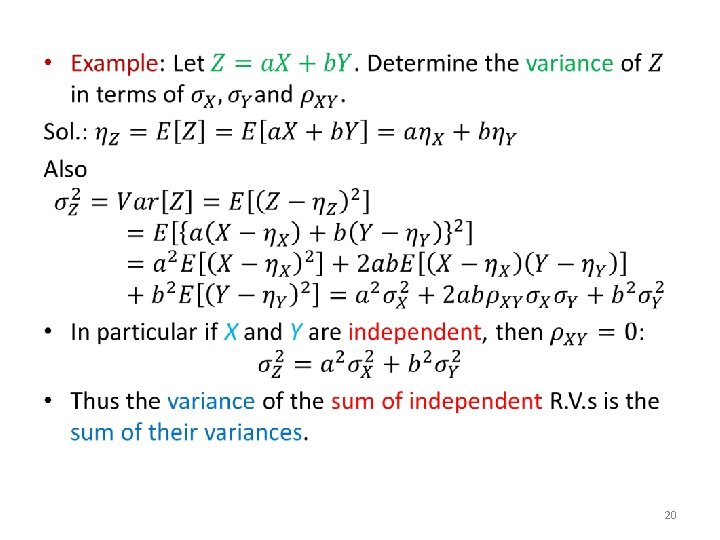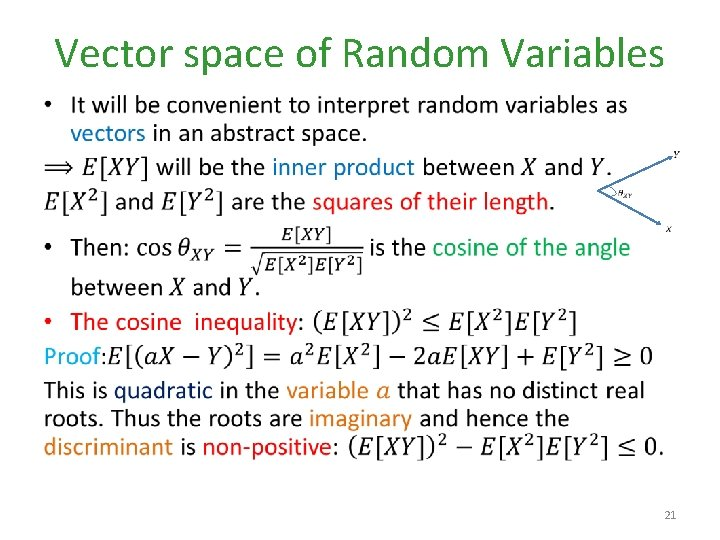Vector space of Random Variables • 21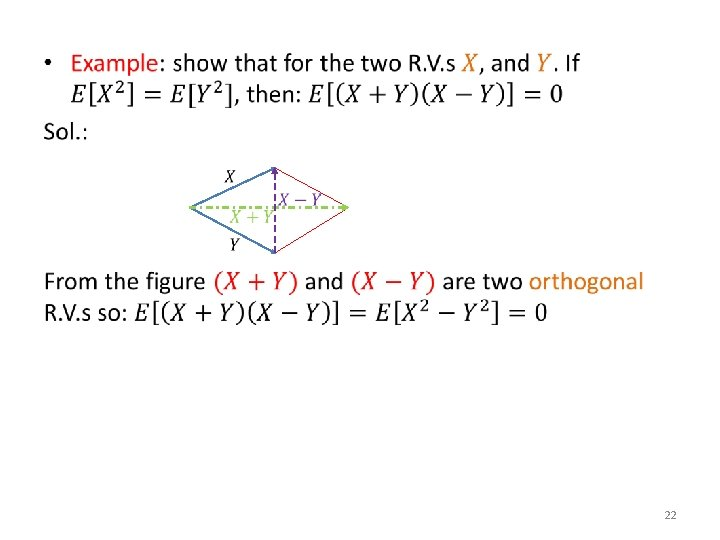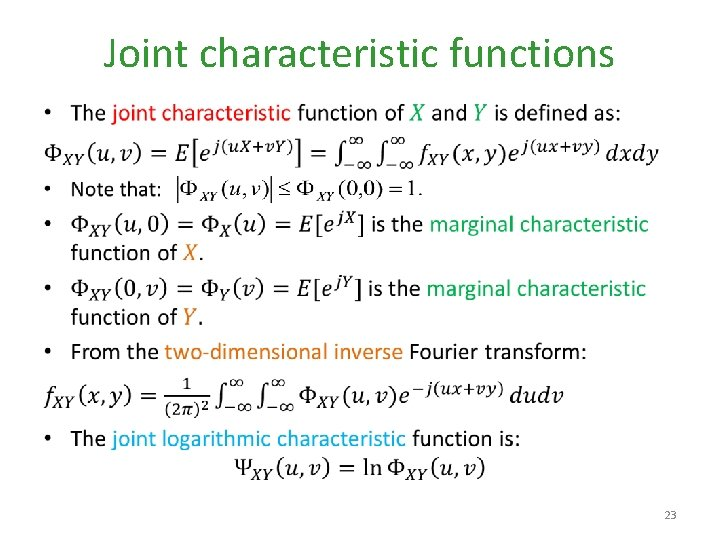Joint characteristic functions • 23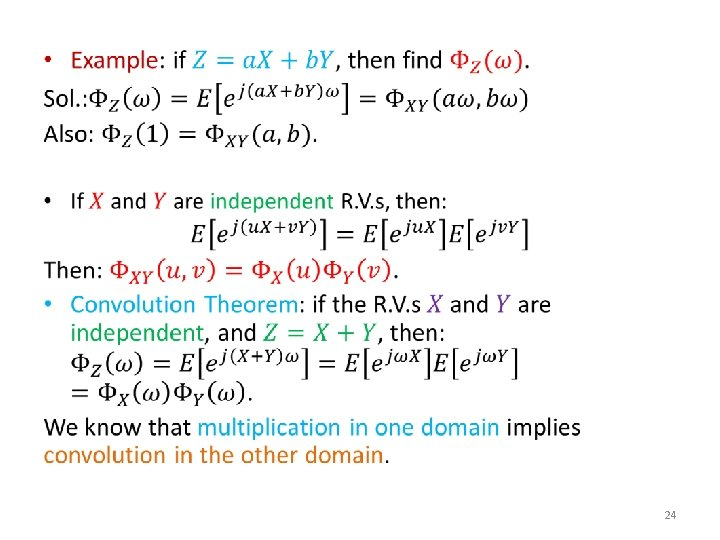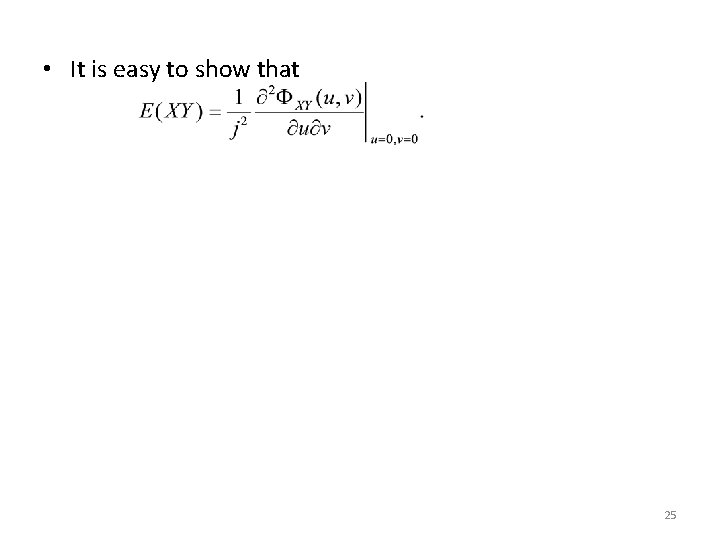• It is easy to show that 25Moment Generating Function • 26More on Gaussian R. V. s • 27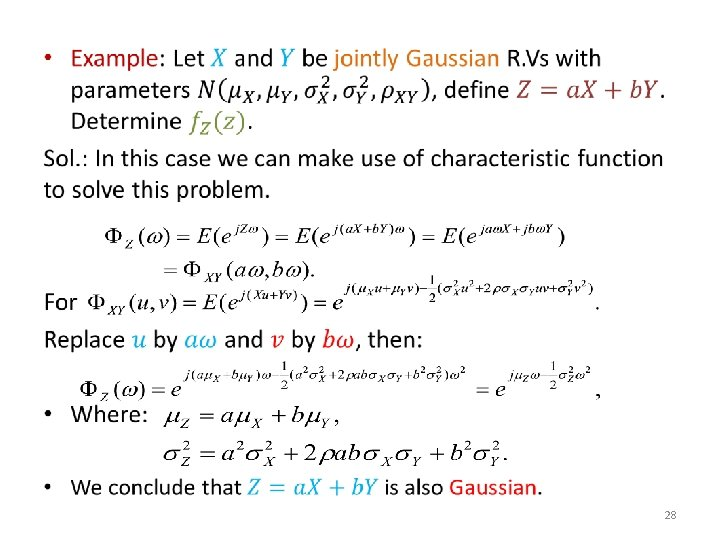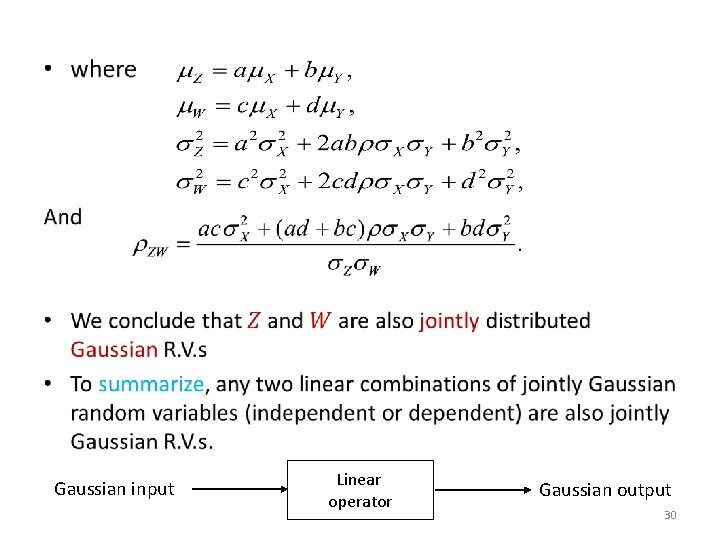• Gaussian input Linear operator Gaussian output 30Central Limit Theorem • 31• we have • Consider: where we have made use of the independence of the R. V. s, but: • Then: and as since 32• In Summary: • The central limit theorem states that a large sum of independent random variables each with finite variance tends to behave like a normal random variable. Thus the individual PDFs become unimportant to analyze the collective sum behavior. • If we model the noise phenomenon as the sum of a large number of independent random variables (e. g. : electron motion in resistor components), then this theorem allows us to conclude that noise behaves like a Gaussian R. V. 33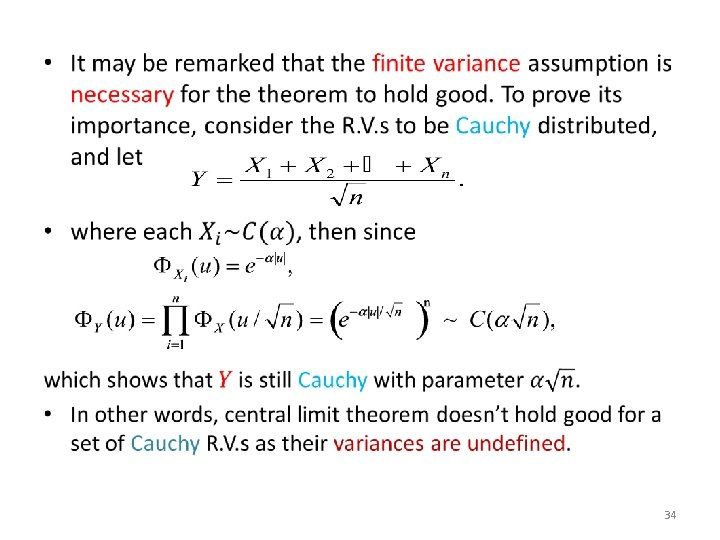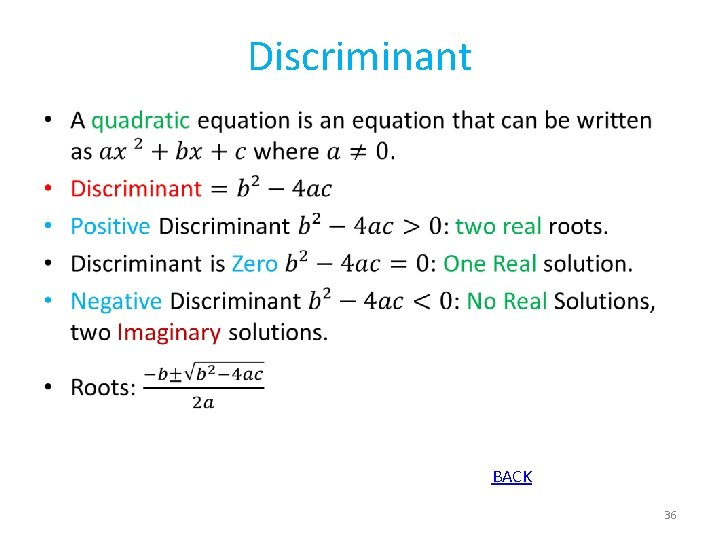Discriminant • BACK 36